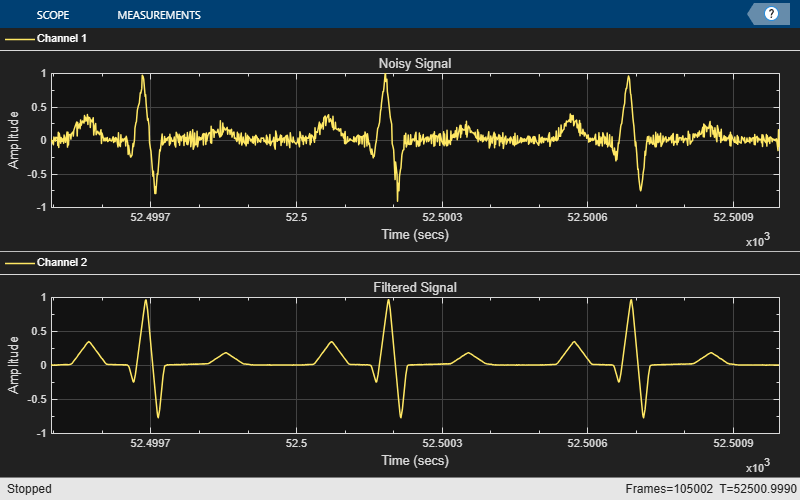## Removing High-Frequency Noise from an ECG Signal

This example shows how to lowpass filter an ECG signal that contains high frequency noise.

Create one period of an ECG signal. The `ecg` function creates an ECG signal of length 500. The `sgolayfilt` function smoothes the ECG signal using a Savitzky-Golay (polynomial) smoothing filter.

```x = ecg(500).'; y = sgolayfilt(x,0,5); [M,N] = size(y); ```

Initialize the time scope to view the noisy signal and the filtered signal.

```Fs = 1000; TS = dsp.TimeScope('SampleRate',Fs,... 'TimeSpan',1.5,... 'YLimits',[-1 1],... 'ShowGrid',true,... 'NumInputPorts',2,... 'LayoutDimensions',[2 1],... 'Title','Noisy and Filtered Signals'); ```

Design a minimum-order lowpass filter with a passband edge frequency of 200 Hz and a stopband edge frequency of 400 Hz. The desired amplitude of the frequency response and the weights are specified in `A` and `D` vectors, respectively. Pass these specification vectors to the `firgr` function to design the filter coefficients. Pass these designed coefficients to the `dsp.FIRFilter` object.

```Fpass = 200; Fstop = 400; Dpass = 0.05; Dstop = 0.0001; F = [0 Fpass Fstop Fs/2]/(Fs/2); A = [1 1 0 0]; D = [Dpass Dstop]; b = firgr('minorder',F,A,D); LP = dsp.FIRFilter('Numerator',b); ```

Design a minimum-order highpass filter with a stopband edge frequency of 200 Hz and a passband edge frequency of 400 Hz. Design the filter using the `firgr` function. Pass these designed coefficients to the `dsp.FIRFilter` object.

```Fstop = 200; Fpass = 400; Dstop = 0.0001; Dpass = 0.05; F = [0 Fstop Fpass Fs/2]/(Fs/2); % Frequency vector A = [0 0 1 1]; % Amplitude vector D = [Dstop Dpass]; % Deviation (ripple) vector b = firgr('minord',F,A,D); HP = dsp.FIRFilter('Numerator',b); ```

The noisy signal contains the smoothed ECG signal along with high frequency noise. The signal is filtered using a lowpass filter. View the noisy signal and the filtered signal using the time scope.

```tic; while toc < 30 x = .1 * randn(M,N); highFreqNoise = HP(x); noisySignal = y + highFreqNoise; filteredSignal = LP(noisySignal); TS(noisySignal,filteredSignal); end % Finalize release(TS) ```Watch now